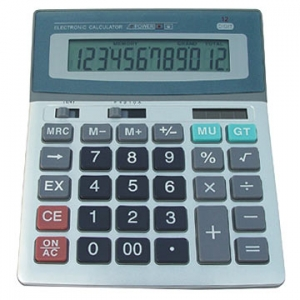Calculators allowed (2)...My cousin started playing with calculator... but he incidentally typed a number "A" and divided it by a number "B" and surprisingly the result came as 0.365065065065...

What is the smallest value of A and B (both are positive integers) which satisfies the above condition. If you got A and B, what is A+B?

Hints and Directions:

1. Calculators are not going to help.
2. Suppose you got 35 and 40, deduce them to 7 and 8 and then add them and give the answer as 15.
×